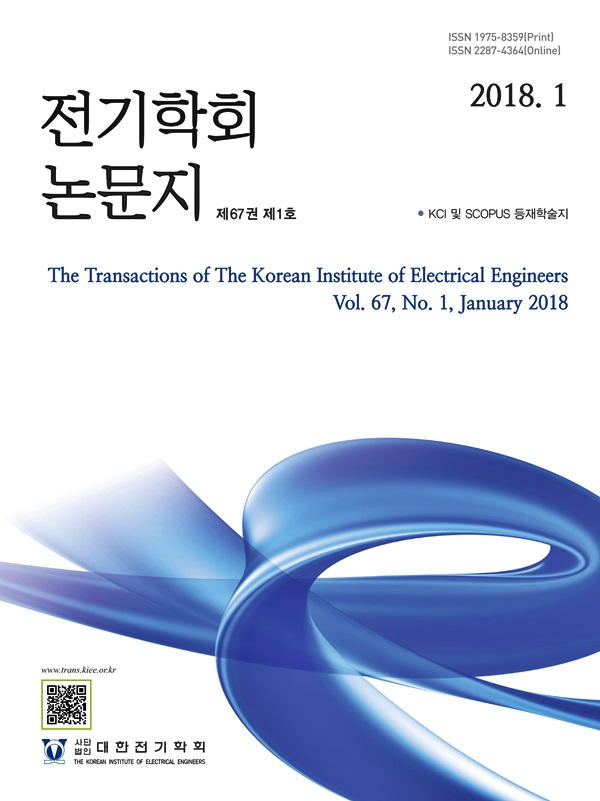Mobile QR Code#### The Transactions of the Korean Institute of Electrical Engineers

##### ISO Journal TitleTrans. Korean. Inst. Elect. Eng.
Title A Methodology on the Optimal Control of Generator Reference Voltage Considering Transient Stability Constraints 서상수(Sangsoo Seo) https://doi.org/10.5370/KIEE.2021.70.12.1786 pp.1786-1791 1975-8359 Generator Voltage Control; Optimal Power Flow; Special Protection System; Transient Stability In this paper, we propose a method to solve the system overvoltage problem without causing the transient stability problem under light load of the power system. The optimal voltage can be found by applying the operating voltage constraints and transient stability constraints to the existing optimization function which the minimizing losses is the objective function. The method of applying the transient stability constraint is divided into two steps. The first is to find the voltage range of the power plant that satisfies the transient stability. Next, the optimal operating voltage is calculated by considering the derived voltage range as the voltage range constraint of the generator of the optimization function. The calculated the optimal reference voltage of generators can solve the system overvoltage problem without impairing the transient stability in case of a power system light load situation. The effect of the proposed scheme was verified using a light load Korean power system